Author: Oscar Cronquist Article last updated on February 21, 2023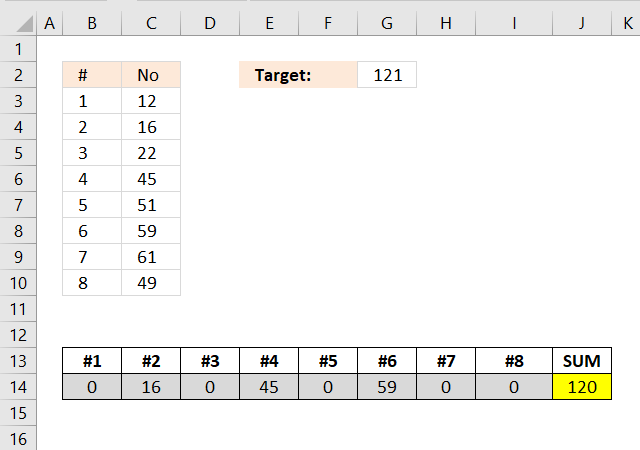Excelxor is such a great website for inspiration, I am really impressed by this post Which numbers add up to total? (2): Multiple Solutions. The MMULT function is used in a really clever way, I thought that function was pretty much useless.

I modified the formula in excelxor's post so it can identify the sum that is nearest to the target value if there isn't a total equal to the target value.

## 1. Find numbers closest to sumThe animated picture shows two target values in cell E1. The result is in cell range E6:L6 and the sum is in cell M6.

Array formula in cell range E6:L6:

=INDEX(MOD(INT((ROW(\$C\$1:INDEX(\$C:\$C, 2^ROWS(\$C\$3:\$C\$10)))-1)/2^(TRANSPOSE(MATCH(ROW(\$C\$3:\$C\$10), ROW(\$C\$3:\$C\$10)))-1)), 2)*TRANSPOSE(\$C\$3:\$C\$10), MATCH(MIN(ABS(MMULT(MOD(INT((ROW(\$C\$1:INDEX(\$C:\$C, 2^ROWS(\$C\$3:\$C\$10)))-1)/2^(TRANSPOSE(MATCH(ROW(\$C\$3:\$C\$10), ROW(\$C\$3:\$C\$10)))-1)), 2), \$C\$3:\$C\$10)-\$G\$2)), ABS(MMULT(MOD(INT((ROW(\$C\$1:INDEX(\$C:\$C, 2^ROWS(\$C\$3:\$C\$10)))-1)/2^(TRANSPOSE(MATCH(ROW(\$C\$3:\$C\$10), ROW(\$C\$3:\$C\$10)))-1)), 2), \$C\$3:\$C\$10)-\$G\$2), 0), 0)

### 1.1 How do I enter this array formula?

1. Select cell range E6:L6
2. Paste the array formula in the formula bar
3. Press an hold CTRL + SHIFT
4. Press Enter
5. Release all keys

If you did it right the formula now begins and ends with curly brackets, like this {=array formula}. They appear automatically, don´t enter the curly brackets yourself.

### 1.2 I want to use this formula, how do I change it?

The array formula uses this cell range \$B\$2:\$B\$9, if you need to work with more values like \$B\$2:\$B\$15 , you can change the formula following these steps.

1. Copy the formula to notepad
2. Press CTRL + H
3. Search string \$B\$2:\$B\$9
4. Replace string \$B\$2:\$B\$15
5. Press with left mouse button on "Replace all" button
6. Copy the formula from notepad
7. Delete the old formula in excel
8. Enter the new array formula in E6:Q6, see steps above.

### 1.3 How many numbers can I use?

If you have more than 15 numbers I recommend using my udf: Excel udf: Find positive and negative amounts that net to zero I think my udf can handle up to 20 maybe 22 values, it depends on your computer hardware.

Torstein made a great comment below: Is it possible to restrict solutions to those which have eg three numbers that adds to the total?
Yes, it is. See his question and my answer with a workbook you can get.

### 1.4 Explaining formula in cell E6

#### Step 1 - Calculate the number of combinations

The ROWS function calculates the number of rows in a cell range.

To calculate the number of combinations we can use the COMBIN function.

COMBIN(8,1) returns 8.

COMBIN(8,2) returns 28.

COMBIN(8,3) returns 56.

COMBIN(8,4) returns 70.

COMBIN(8,5) returns 56.

COMBIN(8,6) returns 28.

COMBIN(8,7) returns 8.

COMBIN(8,8) returns 1.

8+28+56+70+56+28+8+1 = 255

10 numbers return 1024 combinations.

11 numbers return 2048 combinations.

15 numbers return 32768 combinations.

20 numbers return 1048576 combinations. This is the limit, Excel can't handle arrays larger than this number. Note, VBA functions may use much larger arrays.

Instead of using the COMBIN function you can use the exponent character to calculate the number of combinations. 2 ^ 8 = 2*2*2*2*2*2*2*2 = 256. Subtract 256 with 1 and we have the number we are looking for.

INDEX(\$C:\$C, 2^ROWS(\$C\$3:\$C\$10)))

becomes

INDEX(\$C:\$C,2^8)

2^8 is two to the power of 8 meaning 2*2*2*2*2*2*2*2 = 256

becomes

INDEX(\$C:\$C,256)

and returns \$C\$256.

#### Step 2 - Create an array from 1 to 255

The ROW function creates a number for each cell in a cell range.

ROW(\$C\$2:INDEX(\$C:\$C,2^ROWS(\$C\$2:\$C\$9)))-1

becomes

ROW(\$C\$2:\$C\$256)-1

becomes

{2; 3; 4; 5; 6; 7; 8; 9; ... to .... ; 256}-1

and returns

{1; 2; 3; 4; 5; 6; 7; 8; ... to .... ; 255}

This array will be used to create a larger array that shows all combinations.

#### Step 3 - Create combination array

The INT function removes the decimal part from positive numbers and returns the whole number.

INT((ROW(\$C\$3:INDEX(\$C:\$C, 2^ROWS(\$C\$3:\$C\$10)))-1)/2^(TRANSPOSE(MATCH(ROW(\$C\$3:\$C\$10), ROW(\$C\$3:\$C\$10)))-1))

becomes

INT({1; 2; 3; 4; 5; 6; 7; 8; ... to .... ; 255}/2^(TRANSPOSE(MATCH(ROW(\$C\$2:\$C\$9),ROW(\$C\$2:\$C\$9)))-1))

becomes

INT({1; 2; 3; 4; 5; 6; 7; 8; ... to .... ; 255}/2^(TRANSPOSE({1; 2; 3; 4; 5; 6; 7; 8})-1))

becomes

INT({1; 2; 3; 4; 5; 6; 7; 8; ... to .... ; 255}/2^({1, 2, 3, 4, 5, 6, 7, 8}-1))

becomes

INT({1; 2; 3; 4; 5; 6; 7; 8; ... to .... ; 255}/2^({0, 1, 2, 3, 4, 5, 6, 7}))

becomes

INT({1; 2; 3; 4; 5; 6; 7; 8; ... to .... ; 255}/{1, 2, 4, 8, 16, 32, 64, 128})

becomes

INT( {1, 0.5, 0.25, 0.125, 0.0625, 0.03125, 0.015625, 0.0078125; 2, 1, 0.5, ... to ... ; 1.9921875} )

and returns

{1, 0, 0, 0, 0, 0, 0, 0; ... to .... ; 255, 127, 63, 31, 15, 7, 3, 1}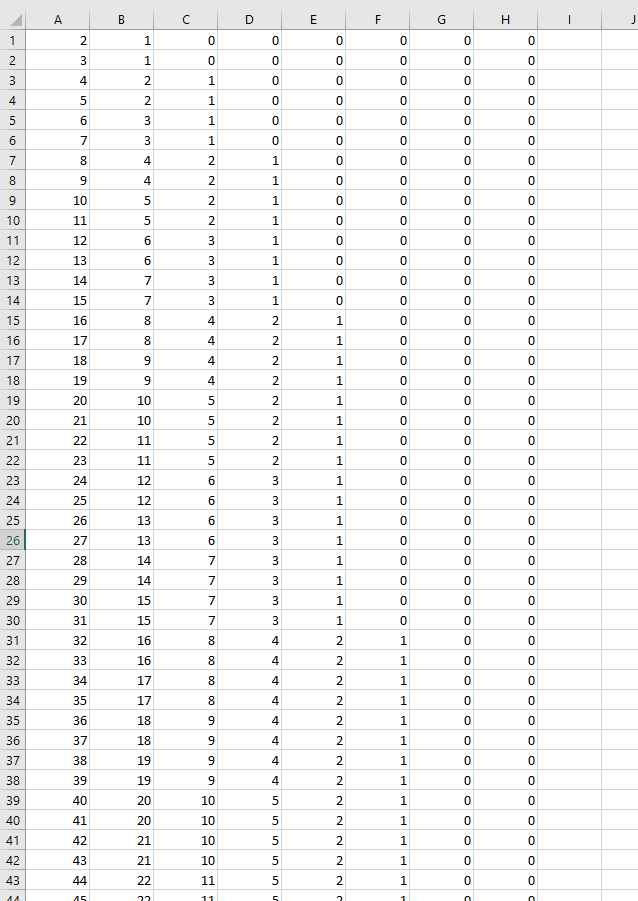This image shows the first 43 rows (out of 255 rows) of the array.

#### Step 4 - Create boolean array

The MOD function returns the remainder after a number is divided by a divisor.  This allows you to create an array that shows which numbers are valid for any given combination.

MOD(INT((ROW(\$C\$3:INDEX(\$C:\$C, 2^ROWS(\$C\$3:\$C\$10)))-1)/2^(TRANSPOSE(MATCH(ROW(\$C\$3:\$C\$10), ROW(\$C\$3:\$C\$10)))-1)),2)

becomes

MOD({1, 0, 0, 0, 0, 0, 0, 0; ... to .... ; 255, 127, 63, 31, 15, 7, 3, 1},2)

and returns

{1,0,0,0,0,0,0,0;... to ... ;1,1,1,1,1,1,1,1}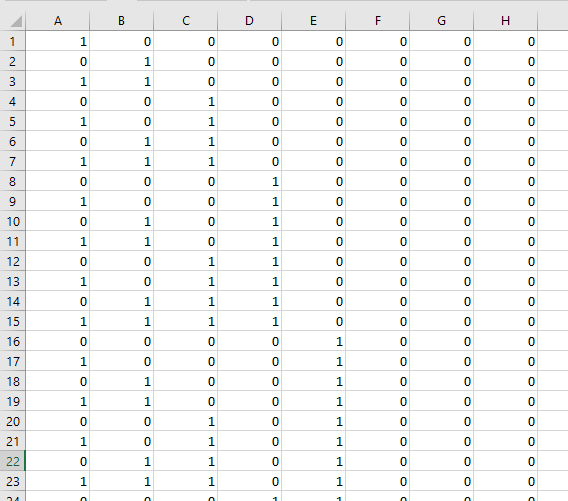#### Step 5 - Find total closest to target value

Calculations in step 1 to 4 are made several times in the formula, it returns an array that contains all possible combinations of the numbers used.

In this example, there are eight numbers. The calculations return an array with 255 rows and 8 columns. 255 corresponds with the number of combinations.

If I replace these calculations with the name array1 the formula becomes:

=INDEX(array1*TRANSPOSE(\$C\$3:\$C\$10), MATCH(MIN(ABS(MMULT(array1, \$C\$3:\$C\$10)-\$G\$2)), ABS(MMULT(array1, \$C\$3:\$C\$10)-\$G\$2), 0), 0)

This makes it easier to understand and troubleshoot the formula. Let start with this part of the formula:

MIN(ABS(MMULT(array1, \$C\$3:\$C\$10)-\$G\$2))

This part of the formula finds the total number that is closest to 0 (zero) meaning that number is closest to the target value. The MMULT function adds numbers on the same row and returns an array containing the totals for each combination.

MIN(ABS(MMULT(array1, \$C\$3:\$C\$10)-\$G\$2))

If we subtract each total with the target value we can use the MIN function to find the total that is closest to target value.

MIN(ABS(MMULT(array1, \$C\$3:\$C\$10)-\$G\$2))

becomes

MIN(ABS({16; 28; 22; 34; 38; 50; 45; 57; 61; 73; ... ; 315}-\$G\$2))

becomes

MIN(ABS({16; 28; 22; 34; 38; 50; 45; 57; 61; 73; ... ; 315}-121))

becomes

MIN({105;93;99;87;83;71;76;64;60;48; ... ;194})

and returns 1.

#### Step 6 - Create array to search

We calculated this array in the previous step, however, we need to calculate this array again in order to find the position of the closest value in the array.

ABS(MMULT(MOD(INT((ROW(\$C\$3:INDEX(\$C:\$C, 2^ROWS(\$C\$3:\$C\$10)))-1)/2^(TRANSPOSE(MATCH(ROW(\$C\$3:\$C\$10), ROW(\$C\$3:\$C\$10)))-1)), 2), \$C\$3:\$C\$10)-\$G\$2)

returns this array:

{105;93;99;87;83;71;76;64;60;48; ... ;194}

#### Step 7 - Find the relative position

The MATCH function returns the relative position of a given number in a cell range or array.

MATCH(MIN(ABS(MMULT(MOD(INT((ROW(\$C\$3:INDEX(\$C:\$C, 2^ROWS(\$C\$3:\$C\$10)))-1)/2^(TRANSPOSE(MATCH(ROW(\$C\$3:\$C\$10), ROW(\$C\$3:\$C\$10)))-1)), 2), \$C\$3:\$C\$10)-\$G\$2)), ABS(MMULT(MOD(INT((ROW(\$C\$3:INDEX(\$C:\$C, 2^ROWS(\$C\$3:\$C\$10)))-1)/2^(TRANSPOSE(MATCH(ROW(\$C\$3:\$C\$10), ROW(\$C\$3:\$C\$10)))-1)), 2), \$C\$3:\$C\$10)-\$G\$2), 0)

becomes

MATCH(1, ABS(MMULT(MOD(INT((ROW(\$C\$3:INDEX(\$C:\$C, 2^ROWS(\$C\$3:\$C\$10)))-1)/2^(TRANSPOSE(MATCH(ROW(\$C\$3:\$C\$10), ROW(\$C\$3:\$C\$10)))-1)), 2), \$C\$3:\$C\$10)-\$G\$2), 0)

becomes

MATCH(1, {105;93;99;87;83;71;76;64;60;48; ... ;194}, 0)

and returns 41.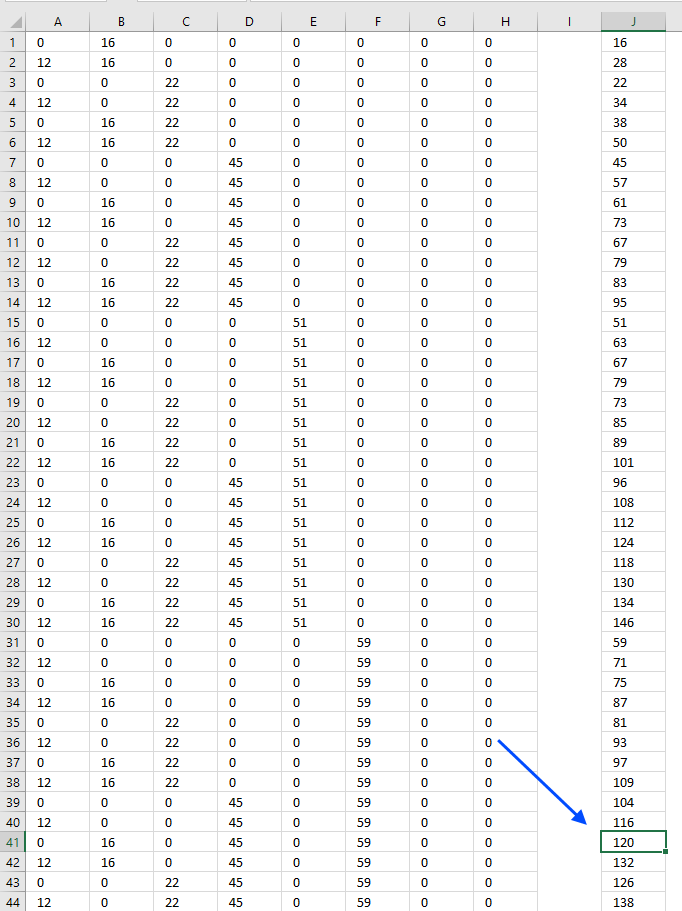Total 120 is closest to target value 121, the chosen numbers are shown on the same row. Now we need to get those numbers and display them on the worksheet.

#### Step 8 - Return values

The INDEX function returns a value or multiple values based on a row and column number. IF 0 (zero) is used the entire row or column is returned.

INDEX(array1*TRANSPOSE(\$C\$3:\$C\$10), MATCH(MIN(ABS(MMULT(array1, \$C\$3:\$C\$10)-\$G\$2)), ABS(MMULT(array1, \$C\$3:\$C\$10)-\$G\$2), 0), 0)

becomes

INDEX(array1*TRANSPOSE(\$C\$3:\$C\$10), 41,  0)

becomes

INDEX(array1*{12;16;22;45;51;59;61;49}, 41,  0)

becomes

INDEX({0, 16, 0, 0, 0, 0, 0, 0;12, 16, ... , 49}, 41,  0)

and returns {0, 16, 0, 45, 0, 59, 0, 0} in cell range B14:I14.## 2. Find numbers closest to sum - Excel 365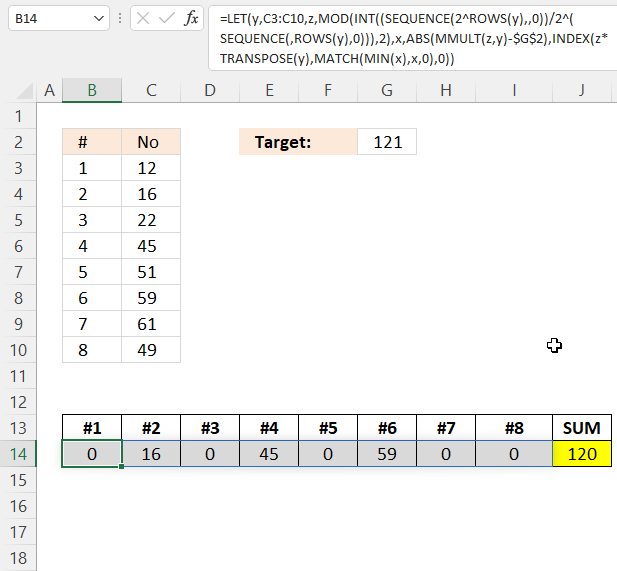The Excel 365 formula is 143 characters long, the earlier formula demonstrated in section 1 above is 458 characters long.

Dynamic array formula in cell B14:

=LET(y,C3:C10,z,MOD(INT((SEQUENCE(2^ROWS(y),,0))/2^(SEQUENCE(,ROWS(y),0))),2),x,ABS(MMULT(z,y)-\$G\$2),INDEX(z*TRANSPOSE(y),MATCH(MIN(x),x,0),0))

It is mostly the LET function that makes this formula less than one-third in size.

## 3. Find numbers in total with a condition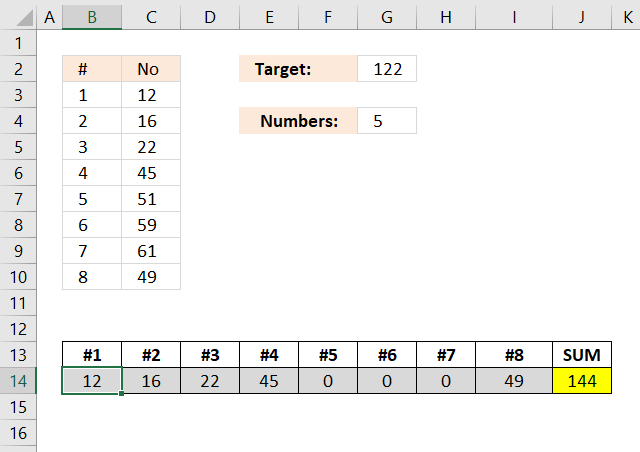Array formula in cell range B14:I14:

=INDEX(MOD(INT((ROW(\$C\$3:INDEX(\$C:\$C, 2^ROWS(\$C\$3:\$C\$10)))-1)/2^(TRANSPOSE(MATCH(ROW(\$C\$3:\$C\$10), ROW(\$C\$3:\$C\$10)))-1)), 2)*TRANSPOSE(\$C\$3:\$C\$10), MATCH(MIN(IF(MMULT(MOD(INT((ROW(\$C\$3:INDEX(\$C:\$C, 2^ROWS(\$C\$3:\$C\$10)))-1)/2^(TRANSPOSE(MATCH(ROW(\$C\$3:\$C\$10), ROW(\$C\$3:\$C\$10)))-1)), 2), C3:C10^0)=\$G\$4, ABS(MMULT(MOD(INT((ROW(\$C\$3:INDEX(\$C:\$C, 2^ROWS(\$C\$3:\$C\$10)))-1)/2^(TRANSPOSE(MATCH(ROW(\$C\$3:\$C\$10), ROW(\$C\$3:\$C\$10)))-1)), 2), \$C\$3:\$C\$10)-\$G\$2), "")), IF(MMULT(MOD(INT((ROW(\$C\$3:INDEX(\$C:\$C, 2^ROWS(\$C\$3:\$C\$10)))-1)/2^(TRANSPOSE(MATCH(ROW(\$C\$3:\$C\$10), ROW(\$C\$3:\$C\$10)))-1)), 2), C3:C10^0)=\$G\$4, ABS(MMULT(MOD(INT((ROW(\$C\$3:INDEX(\$C:\$C, 2^ROWS(\$C\$3:\$C\$10)))-1)/2^(TRANSPOSE(MATCH(ROW(\$C\$3:\$C\$10), ROW(\$C\$3:\$C\$10)))-1)), 2), \$C\$3:\$C\$10)-\$G\$2), ""), 0), 0)

### 3.1 Explaining formula in cell B14

I explained the previous formula above and this formula is very similar, however, it is easier to understand the formula if I replace some calculations that are repeated several times in the formula with array1.

=INDEX(array1*TRANSPOSE(\$C\$3:\$C\$10), MATCH(MIN(IF(MMULT(array1, C3:C10^0)=\$G\$4, ABS(MMULT(array1, \$C\$3:\$C\$10)-\$G\$2), "")), IF(MMULT(array1, C3:C10^0)=\$G\$4, ABS(MMULT(array1, \$C\$3:\$C\$10)-\$G\$2), ""), 0), 0)

array1 -> MOD(INT((ROW(\$C\$3:INDEX(\$C:\$C, 2^ROWS(\$C\$3:\$C\$10)))-1)/2^(TRANSPOSE(MATCH(ROW(\$C\$3:\$C\$10), ROW(\$C\$3:\$C\$10)))-1)), 2)

This formula filters rows in the array that only have the same number of items as the specified value in cell G4. The following IF function filters rows that contain the same number of items as the target value:

IF(MMULT(array1, C3:C10^0)=\$G\$4, ABS(MMULT(array1, \$C\$3:\$C\$10)-\$G\$2), "")

## 4. Find numbers in total with a condition - Excel 365The image above demonstrates a formula that lets you calculate the closest target sum specified in cell G2 and how many numbers specified in cell G4.

The formula in cell B14 calculates which numbers to use, specified in cell range C3:C10, to get as close as possible to the target value using a given number of numbers.

Cell G2 contains 122 and that is the target sum, cell G4 contains 5 which is how many numbers in cells C3:C10 the formula can use. The result in cells B14:I14 show a total of 5 numbers and the sum is shown in cell J14 which is 144. This is the closest you can get to 122 using only five numbers.

Excel 365 dynamic array formula in cell B14:

=LET(z,C3:C10,q,ROW(z),x,MOD(INT((ROW(\$C\$3:INDEX(\$C:\$C, 2^ROWS(z)))-1)/2^(TRANSPOSE(MATCH(q, q))-1)), 2),y,ABS(MMULT(x, z)-\$G\$2),w,IF(MMULT(x, z^0)=\$G\$4, y, ""),INDEX(x*TRANSPOSE(z), MATCH(MIN(w), w, 0), 0))

The new Excel 365 LET function allows me to create a much shorter formula than the one in section 3. The formula is basically the same, however, parts that are repeated are shortened in order to improve calculations and create a smaller formula.

The LET function lets you name intermediate calculation results which can shorten formulas considerably and improve performance.

Function syntax: LET(name1, name_value1, calculation_or_name2, [name_value2, calculation_or_name3...])

Which numbers add up to total? (2): Multiple Solutions

Which numbers add up to total?

Excel udf: Find positive and negative amounts that net to zero

Identify numbers in sum using solver

Find positive and negative amounts that net to zero

### Get the Excel fileFind-numbers-which-are-closest-to-a-total.xlsx